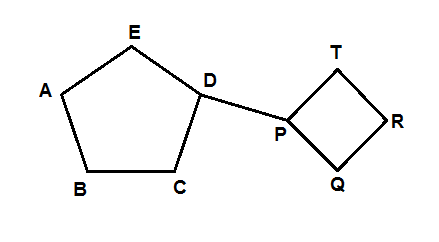# The tutor defines cut set with a couple of examples.

When one imagines a cut set, one imagines removing edges from a graph, but not the vertices they connect.

In this context, we are thinking of undirected graphs.

A cut set is the minimum number of edges whose removal will disconnect a given portion of the graph. Each cut set is specific to a portion of the graph to be disconnected.

Consider the graph below:To disconnect just the vertex A from the graph, edges AB and AE need to be removed. The corresponding cut set is {AE, AB}. This may also be written {{A,E},{A,B}}, meaning “[the set containing] the edge from A to E or E to A, and the edge from A to B or B to A”.

{DP}, or {{D,P}}, is also a cut set: removing the edge DP separates the two cyclic portions of the graph.

When we disconnect one part of a graph from the rest, we say the number of components of the graph has increased by 1. A graph’s number of components is its number of isolated parts. Before any cut sets are applied, a connected graph (such as the one above) has 1 component: κ(graph)=1. A component, within itself, can be called a connected component.

HTH:)

Source:

Grimaldi, Ralph P. Discrete and Combinatorial Mathematics. Don Mills: Addison-
Wesley, 1994.

Jack of Oracle Tutoring by Jack and Diane, Campbell River, BC.

Tagged with: , ,